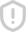### Introduction to Modern Cryptography - Jonathan Katz & Yehuda Lindell 评分Introduction to Modern Cryptography - Jonathan Katz & Yehuda Lindell

...展开详情举报收藏分享Introduction to Modern Cryptography 2nd 立即下载Introduction to Modern Cryptography 立即下载Introduction to Modern Cryptography, Second Edition 立即下载Introduction to Modern Cryptography 2ed -- by Jonathan Katz and Yehuda Lindell 立即下载Introduction to modern cryptography by jonathan katz & yehuda lindell 立即下载introduction to modern cryptography.pdf 立即下载Jonathan Katz 密码学ppt 立即下载Introduction to Modern Cryptography.pdf 立即下载html+css+js制作的一个动态的新年贺卡 立即下载Camtasia 9安装及破解方法绝对有效 立即下载rar.zip.7z密码破解 立即下载lonerloner